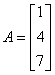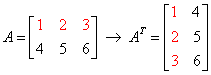# Matrix Algebra Assignment Help, Types of Matrices, Matrix Mathematics Help

### Math Assignment Help >> Matrix AlgebraLive Math Experts: Defining Matrix, Types of Matrices, Matrix Algebra, Math Assignment Help In mathematics, a matrix is a rectangular array of numbers, expressions or symbols. The individual items in a matrix are known its elements or entries. There are various applications of matrices in most scientific fields. In physics, matrices are generally used to study optics, electrical circuits and quantum mechanics. In computer graphics, matrices are mostly used to project a 3-dimensional image onto a 2-dimensional screen and to create realistic seeming motion. Matrix calculus generalizes the classical analytical notions for example exponentials to higher dimensions and derivatives. Matrix algebra is very interesting subjects and its applications are using in most of the fields including physics and some analytical subjects. Thus the idea of matrix calculations are essential for students, not for studying mathematics but in other filed like physics, mechanics and other calculations. Sometimes students stuck with matrix or different types of matrices problems and assignments. Matrix algebra problems are not quite easy; some of complex matrix algebra problems cannot be done without expert’s assistance. Math experts at Expertsmind.com are working for students in solving their doubts and problems facing in their studies. The student, who feels trouble in solving their matrix algebra problem, can ask question anytime from anywhere at Expertsmind.com from qualified and experienced math experts. Our mathematics experts are highly qualified and experienced and they have ability to solve your toughest math problems in easy way. They offer you matrix algebra solutions with step by step answers. Type of Matrices 1. Square Matrix: A matrix is called as square matrix if it has same number of columns and rows. For example if a matrix has n columns and n rows, then it is known as square matrix.2. Diagonal Matrix: A diagonal matrix is square matrixes which all elements are zero excluding diagonal elements. Thus diagonal matrix is completely denoted by its diagonal elements. In given example diagonal matrix diag(1 , 5 , 9) is defined as given below:3. Row Matrix: A matrix which has only one row is called row matrix. For example C=[2, 5, 7,8] 4. Column Matrix: A matrix which has only one column is known as column matrix for example5. Transpose of a Matrix: If A matrix is defined as n*m then transpose matrix of A is defined as AT such as m*n matrix6. Zero Matrix: A matrix is called zero matrix if all of its elements are zero.7. Identity Matrix I: Identity matrix is a diagonal matrix which all diagonal elements are equal to 1.8. Opposite Matrix: A matrix opposite matrix –A defined as if all elements sign is changes.9. Symmetric Matrix: A matrix is known as symmetric matrix if it is equal to its transpose matrix.# Why Us ?